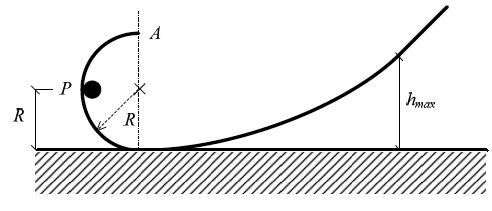# Launching A Punctiform Object

Consider a circular path of radius $R$, when passing through the point $P$ the magnitude of the resultant force on the object is $\sqrt{17}\text{ mg}$, where $g$ is the acceleration of gravity.

Find the maximum height $h_{\text{max}}$ that the object reaches the ramp in terms of $R$.×

Problem Loading...

Note Loading...

Set Loading...